﻿ 基于改进精英势场蚁群算法的机器人三维路径规划算法研究

# 基于改进精英势场蚁群算法的机器人三维路径规划算法研究Robot 3D Path Planning Algorithm Based on Improved Elitist Potential Field Ant Colony Algorithm

Abstract: Three dimensional path planning is an important part of robot to complete the target task. Aiming at the problems of slow convergence speed, easy to fall into local optimal solution and low smoothness in the later stage of ant colony algorithm, therefore this paper proposes a robot 3D path planning algorithm based on improved elitist potential field ant colony algorithm. Firstly, the orientation of the artificial potential field is introduced to initialize the pheromone concentration to accelerate the convergence speed of the algorithm, and the pheromone concentration update method based on elite strategy is adopted to enhance the guidance of excellent individuals to the population, and the two-way search strategy is adopted to further accelerate the convergence speed of the algorithm and avoid the algorithm falling into the local optimal solution, and then the direction expansion search strategy is introduced to improve the trajectory Line processing to improve smoothness. Through simulation experiments, the elitist potential field ant colony algorithm, ant colony algorithm and particle swarm algorithm are compared. The experimental results show that compared with other intelligent optimization algorithms, the elitist potential field ant colony algorithm has fast convergence speed, high search efficiency, better and smoother final search path.

1. 引言

2. 蚁群算法

${P}_{ij}\left(t\right)=\left\{\begin{array}{l}\frac{{\left[{\tau }_{ij}\left(t\right)\right]}^{\alpha }{\left[{\eta }_{ij}\left(t\right)\right]}^{\beta }}{\underset{j\in A}{\sum }{\left[{\tau }_{ij}\left(t\right)\right]}^{\alpha }{\left[{\eta }_{ij}\left(t\right)\right]}^{\beta }}\text{ }\text{ }\text{ }\text{ }\text{ }\text{ }\text{ }\text{ }\text{ }\text{ }\text{ }\text{ }\text{ }\text{ }\text{ }\text{ }\text{ }\text{ }\text{ }\text{ }\text{ }\text{ }\text{ }j\in A\\ 0\text{ }\text{ }\text{ }\text{ }\text{ }\text{ }\text{ }\text{ }\text{ }\text{ }\text{ }\text{ }\text{ }\text{ }\text{ }\text{ }\text{ }\text{ }\text{ }\text{ }\text{ }\text{ }\text{ }\text{ }\text{ }\text{ }\text{ }\text{ }\text{ }\text{ }\text{ }\text{ }\text{ }\text{ }\text{ }\text{ }\text{ }\text{ }\text{ }\text{ }\text{ }\text{ }\text{ }\text{ }\text{ }\text{ }\text{ }\text{ }\text{ }\text{ }\text{ }\text{ }\text{ }\text{ }\text{ }\text{ }\text{ }\text{ }\text{ }\text{ }\text{ }\text{ }\text{ }\text{ }\text{ }\text{ }\text{ }\text{ }\text{ }\text{ }\text{ }\text{ }\text{ }\text{ }\text{ }\text{ }\text{ }\text{ }\text{ }\text{ }\text{ }\text{ }\text{ }\text{ }\text{ }\text{ }\text{ }\text{ }\text{ }\text{ }\text{ }\text{ }\text{ }\text{ }\text{ }\text{ }\text{ }\text{ }\text{ }\text{ }\text{ }\text{ }\text{ }\text{ }\text{ }\text{ }其他\end{array}$ (1)

${\eta }_{ij}\left(t\right)=\frac{1}{{L}_{ij}}$ (2)

${\tau }_{ij}\left(t+1\right)=\left(1-\lambda \right){\tau }_{ij}\left(t\right)+\Delta {\tau }_{ij}$ (3)

$\Delta {\tau }_{ij}\left(t\right)=\left\{\begin{array}{l}\frac{1}{{L}_{k}}\text{ }\text{ }\text{ }\text{ }\text{ }\text{ }\text{ }\text{ }\text{ }\text{ }\text{ }\text{ }\text{ }\text{ }\text{ }\text{ }\text{ }\text{ }\text{ }\text{ }\text{ }\text{ }\text{ }{L}_{ij}\in {P}_{k}\\ 0\text{ }\text{ }\text{ }\text{ }\text{ }\text{ }\text{ }\text{ }\text{ }\text{ }\text{ }\text{ }\text{ }\text{ }\text{ }\text{ }\text{ }\text{ }\text{ }\text{ }\text{ }\text{ }\text{ }\text{ }\text{ }\text{ }\text{ }\text{ }\text{ }\text{ }\text{ }\text{ }其他\end{array}$ (4)

3. 人工势场算法

${U}_{att}=\frac{1}{2}k\left(S-E\right)$ (5)

${F}_{att}=-grad\left({U}_{att}\right)=-k\left(S-E\right)$ (6)

${U}_{rep}=\left\{\begin{array}{l}\frac{1}{2}\theta {\left(\frac{1}{\rho }-\frac{1}{{\rho }_{0}}\right)}^{2}\text{ }\text{ }\text{ }\text{ }\text{ }\text{ }\text{ }\text{ }\text{ }\text{ }\text{ }\text{ }\text{ }\text{ }\text{ }\text{ }\text{ }\rho \le {\rho }_{0}\\ 0\text{ }\text{ }\text{ }\text{ }\text{ }\text{ }\text{ }\text{ }\text{ }\text{ }\text{ }\text{ }\text{ }\text{ }\text{ }\text{ }\text{ }\text{ }\text{ }\text{ }\text{ }\text{ }\text{ }\text{ }\text{ }\text{ }\text{ }\text{ }\text{ }\text{ }\text{ }\text{ }\text{ }\text{ }\text{ }\text{ }\text{ }\text{ }\text{ }\text{ }\text{ }\text{ }\text{ }\text{ }\text{ }\text{ }\text{ }\text{ }\text{ }\text{ }\text{ }\text{ }\text{ }\text{ }\text{ }\text{ }\text{ }\text{ }\text{ }\text{ }\text{ }\text{ }\text{ }\text{ }\text{ }\text{ }\text{ }\text{ }\text{ }\text{ }\text{ }\rho >{\rho }_{0}\end{array}$ (7)

${F}_{rep}=-grad\left({U}_{rep}\right)=\left\{\begin{array}{l}\theta \left(\frac{1}{\rho }-\frac{1}{{\rho }_{0}}\right)\frac{1}{{\rho }^{2}}\text{ }\text{ }\text{ }\text{ }\text{ }\text{ }\text{ }\text{ }\text{ }\text{ }\rho \le {\rho }_{0}\\ 0\text{ }\text{ }\text{ }\text{ }\text{ }\text{ }\text{ }\text{ }\text{ }\text{ }\text{ }\text{ }\text{ }\text{ }\text{ }\text{ }\text{ }\text{ }\text{ }\text{ }\text{ }\text{ }\text{ }\text{ }\text{ }\text{ }\text{ }\text{ }\text{ }\text{ }\text{ }\text{ }\text{ }\text{ }\text{ }\text{ }\text{ }\text{ }\text{ }\text{ }\text{ }\text{ }\text{ }\text{ }\text{ }\text{ }\text{ }\text{ }\text{ }\text{ }\text{ }\text{ }\text{ }\text{ }\text{ }\text{ }\text{ }\text{ }\text{ }\text{ }\text{ }\text{ }\text{ }\text{ }\text{ }\text{ }\rho >{\rho }_{0}\end{array}$ (8)

${F}_{tot}={F}_{att}+{F}_{rep}=\left\{\begin{array}{l}\theta \left(\frac{1}{\rho }-\frac{1}{{\rho }_{0}}\right)\frac{1}{{\rho }^{2}}-k\left(S-E\right)\text{ }\text{ }\text{ }\text{ }\text{ }\text{ }\text{ }\text{ }\text{ }\text{ }\text{ }\text{ }\text{ }\rho \le {\rho }_{0}\\ 0\text{ }\text{ }\text{ }\text{ }\text{ }\text{ }\text{ }\text{ }\text{ }\text{ }\text{ }\text{ }\text{ }\text{ }\text{ }\text{ }\text{ }\text{ }\text{ }\text{ }\text{ }\text{ }\text{ }\text{ }\text{ }\text{ }\text{ }\text{ }\text{ }\text{ }\text{ }\text{ }\text{ }\text{ }\text{ }\text{ }\text{ }\text{ }\text{ }\text{ }\text{ }\text{ }\text{ }\text{ }\text{ }\text{ }\text{ }\text{ }\text{ }\text{ }\text{ }\text{ }\text{ }\text{ }\text{ }\text{ }\text{ }\text{ }\text{ }\text{ }\text{ }\text{ }\text{ }\text{ }\text{ }\text{ }\text{ }\text{ }\text{ }\text{ }\text{ }\text{ }\text{ }\text{ }\text{ }\text{ }\text{ }\text{ }\text{ }\text{ }\text{ }\text{ }\text{ }\text{ }\text{ }\text{ }\text{ }\text{ }\text{ }\text{ }\text{ }\text{ }\text{ }\text{ }\text{ }\text{ }\text{ }\text{ }\text{ }\text{ }\text{ }\text{ }\text{ }\text{ }\text{ }\text{ }\text{ }\text{ }\text{ }\text{ }\text{ }\text{ }\rho >{\rho }_{0}\end{array}$ (9)

4. 精英势场蚁群算法

4.1. 精英策略

${\tau }_{ij}\left(t+1\right)=\left(1-\lambda \right){\tau }_{ij}\left(t\right)+\Delta {\tau }_{ij}+{\left(\Delta {\tau }_{ij}\right)}^{+}$ (10)

${\left(\Delta {\tau }_{ij}\right)}^{\text{+}}=\left\{\begin{array}{l}{\left(\frac{it}{i{t}_{\mathrm{max}}}\right)}^{3}×\frac{1}{{L}_{k}}\text{ }\text{ }\text{ }\text{ }\text{ }\text{ }\text{ }\text{ }\text{ }\text{ }k是精英个体\\ 0\text{ }\text{ }\text{ }\text{ }\text{ }\text{ }\text{ }\text{ }\text{ }\text{ }\text{ }\text{ }\text{ }\text{ }\text{ }\text{ }\text{ }\text{ }\text{ }\text{ }\text{ }\text{ }\text{ }\text{ }\text{ }\text{ }\text{ }\text{ }\text{ }\text{ }\text{ }\text{ }\text{ }\text{ }\text{ }\text{ }\text{ }\text{ }\text{ }\text{ }\text{ }\text{ }\text{ }\text{ }\text{ }\text{ }\text{ }\text{ }\text{ }\text{ }\text{ }\text{ }\text{ }\text{ }\text{ }\text{ }\text{ }k非精英个体\end{array}$ (11)

4.2. 双向搜索策略

4.3. 人工势场初始化信息素浓度

${{U}^{\prime }}_{rep}=\left\{\begin{array}{l}\frac{1}{2}\theta \cdot \left(z-{z}_{0}\right)\cdot {\left(\frac{1}{\rho }-\frac{1}{{\rho }_{0}}\right)}^{2}\text{ }\text{ }\text{ }\text{ }\text{ }\text{ }\text{ }\text{ }\text{ }\text{ }\text{ }\text{ }\text{ }\text{ }\text{ }\text{ }\text{ }\rho \le {\rho }_{0}||z>{z}_{0}\\ 0\text{ }\text{ }\text{ }\text{ }\text{ }\text{ }\text{ }\text{ }\text{ }\text{ }\text{ }\text{ }\text{ }\text{ }\text{ }\text{ }\text{ }\text{ }\text{ }\text{ }\text{ }\text{ }\text{ }\text{ }\text{ }\text{ }\text{ }\text{ }\text{ }\text{ }\text{ }\text{ }\text{ }\text{ }\text{ }\text{ }\text{ }\text{ }\text{ }\text{ }\text{ }\text{ }\text{ }\text{ }\text{ }\text{ }\text{ }\text{ }\text{ }\text{ }\text{ }\text{ }\text{ }\text{ }\text{ }\text{ }\text{ }\text{ }\text{ }\text{ }\text{ }\text{ }\text{ }\text{ }\text{ }\text{ }\text{ }\text{ }\text{ }\text{ }\text{ }\text{ }\text{ }\text{ }\text{ }\text{ }\text{ }\text{ }\text{ }\text{ }\text{ }\text{ }\text{ }\text{ }\text{ }\text{ }\text{ }\text{ }\text{ }\text{ }\text{ }\text{ }\text{ }\text{ }\text{ }\text{ }\text{ }\text{ }\text{ }\text{ }\text{ }\text{ }\text{ }\text{ }\text{ }\text{ }\text{ }\text{ }\text{ }\rho >{\rho }_{0}||z\le {z}_{0}\end{array}$ (12)

${{F}^{\prime }}_{rep}=-grad\left({{U}^{\prime }}_{rep}\right)=\left\{\begin{array}{l}\theta \cdot \left(z-{z}_{0}\right)\cdot \left(\frac{1}{\rho }-\frac{1}{{\rho }_{0}}\right)\frac{1}{{\rho }^{2}}\text{ }\text{ }\text{ }\text{ }\text{ }\text{ }\text{ }\text{ }\text{ }\text{ }\text{ }\text{ }\text{ }\text{ }\text{ }\text{ }\text{ }\text{ }\rho \le {\rho }_{0}||z>{z}_{0}\\ 0\text{ }\text{ }\text{ }\text{ }\text{ }\text{ }\text{ }\text{ }\text{ }\text{ }\text{ }\text{ }\text{ }\text{ }\text{ }\text{ }\text{ }\text{ }\text{ }\text{ }\text{ }\text{ }\text{ }\text{ }\text{ }\text{ }\text{ }\text{ }\text{ }\text{ }\text{ }\text{ }\text{ }\text{ }\text{ }\text{ }\text{ }\text{ }\text{ }\text{ }\text{ }\text{ }\text{ }\text{ }\text{ }\text{ }\text{ }\text{ }\text{ }\text{ }\text{ }\text{ }\text{ }\text{ }\text{ }\text{ }\text{ }\text{ }\text{ }\text{ }\text{ }\text{ }\text{ }\text{ }\text{ }\text{ }\text{ }\text{ }\text{ }\text{ }\text{ }\text{ }\text{ }\text{ }\text{ }\text{ }\text{ }\text{ }\text{ }\text{ }\text{ }\text{ }\text{ }\text{ }\text{ }\text{ }\text{ }\text{ }\text{ }\text{ }\text{ }\text{ }\text{ }\text{ }\text{ }\text{ }\text{ }\text{ }\text{ }\text{ }\text{ }\text{ }\text{ }\text{ }\text{ }\text{ }\text{ }\text{ }\text{ }\text{ }\text{ }\text{ }\rho >{\rho }_{0}||z\le {z}_{0}\end{array}$ (13)

${\tau }_{0}\left(x,y,z\right)={{F}^{\prime }}_{tot}\left(x,y,z\right)\cdot {\tau }_{0}$ (14)

4.4. 方向扩展搜索策略

4.5. 基于人工势场的启发信息改进

${\eta }_{ij}\left(t\right)={\left(\frac{i{t}_{\mathrm{max}}-it}{i{t}_{\mathrm{max}}}\right)}^{3}×{a}^{{F}_{tot}\cdot \mathrm{cos}\phi }×\frac{1}{{d}_{ij}}$ (12)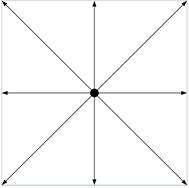(a)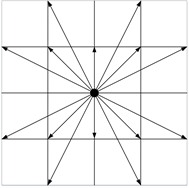(b)

Figure 1. Comparison of individual search direction strategies of ant colony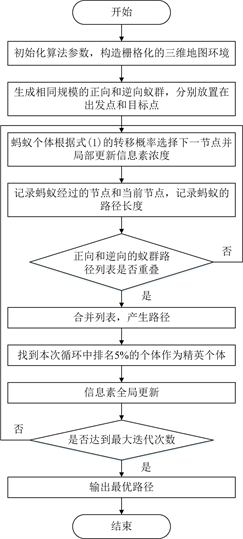Figure 2. Flow chart of improved elitist potential field ant colony algorithm

(1) 初始化算法参数，输入三维地图环境，并对环境进行栅格化建模；

(2) 创建相同规模正向和逆向两个蚂蚁种群，分别置于起点和终点，对每个蚂蚁个体建立节点记录列表；

(3) 算法开始，蚂蚁按照改进后的启发信息和转移概率选择下一节点，并实时更新信息素浓度；

(4) 将选择的节点加入对应的蚂蚁个体节点记录列表中，并记录路径长度；

(5) 如果正向蚁群R1中的某个体的节点记录列表与逆向蚁群R2中的某个体的节点记录列表产生重叠，则在该节点处，将两个列表合并，进而产生一条从出发点向目标点的可行路径，并锁定两只蚂蚁不再继续运动；否则返回步骤(3)；

(6) 找出精英个体，并更新全局信息素；

(7) 判断迭代是否结束，是则输出最优路径；否则返回(3)。

5. 仿真实验研究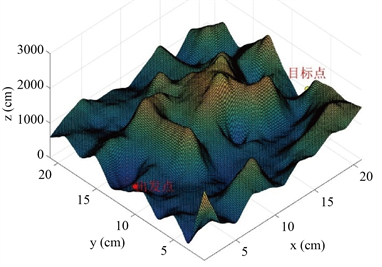Figure 3. Schematic diagram of 3D map environmentTable 1. Parameter setting of elitist potential field ant colony algorithm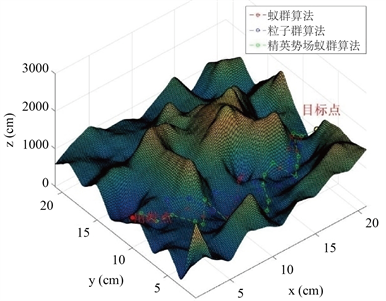(a)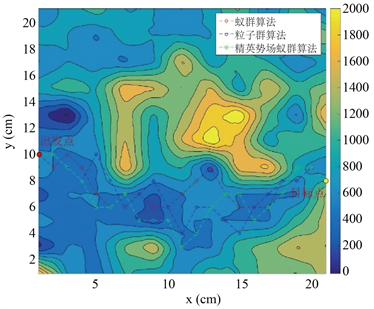(b)

Figure 4. Schematic diagram of path planning results of three algorithmsTable 2. Comparison of path length results of three algorithms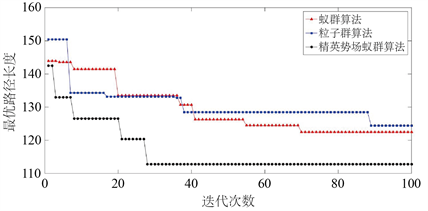Figure 5. Variation curve of optimal path with iteration times

$\text{Gap}=\frac{参{数}_{精英势场蚁群}-参{数}_{蚁群}}{参{数}_{蚁群}}×100%$ (13)Table 3. Comparison results of three algorithms

6. 结论

NOTES

*通讯作者。

 Han, J. (2019) An Efficient Approach to 3D Path Planning. Information Sciences, 478, 318-330.
https://doi.org/10.1016/j.ins.2018.11.045

 Jain, G., Yadav, G., Prakash, D., Shukla, A. and Tiwari, R. (2019) MVO-Based Path Planning Scheme with Coordination of UAVs in 3-D Environment. Journal of Computational Science, 37, Article ID: 101016.
https://doi.org/10.1016/j.jocs.2019.07.003

 姜辰凯, 李智, 盘书宝, 王勇军. 基于改进Dijkstra算法的AGVs无碰撞路径规划[J]. 计算机科学, 2020, 47(8): 272-277.

 李世国, 苏卫华, 郭鹏飞, 张世月, 谢鹏发. 基于改进A~*算法的无人搜救全局路径规划研究[J]. 医疗卫生装备, 2020, 41(12): 16-20.

 刘亚秋, 赵汉琛, 刘勋, 徐妍. 一种改进RRT的工业机器人路径避障规划算法[J/OL]. 信息与控制, 1-13.
https://doi.org/10.13976/j.cnki.xk.2021.0259, 2021-04-12.

 汤迪. 基于改进的人工势场法和水流法的移动机器人三维路径规划[D]: [硕士学位论文]. 中国矿业大学, 2019.

 付兴武, 胡洋. 基于改进粒子群算法的三维路径规划[J/OL]. 电光与控制, 1-5. http://kns.cnki.net/kcms/detail/41.1227.TN.20201201.1055.016.html, 2021-04-12.

 陈超, 张莉. 基于改进蚁群算法的三维路径规划[J]. 计算机工程与应用, 2019, 55(20): 192-196.

 Dorigo, M., Maniezzo, V. and Colorni, A. (1991) Positive Feedback as a Search Strategy. Technical Report, No. 91-016.

 梁献霞, 刘朝英, 宋雪玲, 张英坤. 改进人工势场法的移动机器人路径规划研究[J]. 计算机仿真, 2018, 35(4): 291-294+361.

Top